##### Pre-Calculus: 1001 Practice Problems For Dummies (+ Free Online Practice)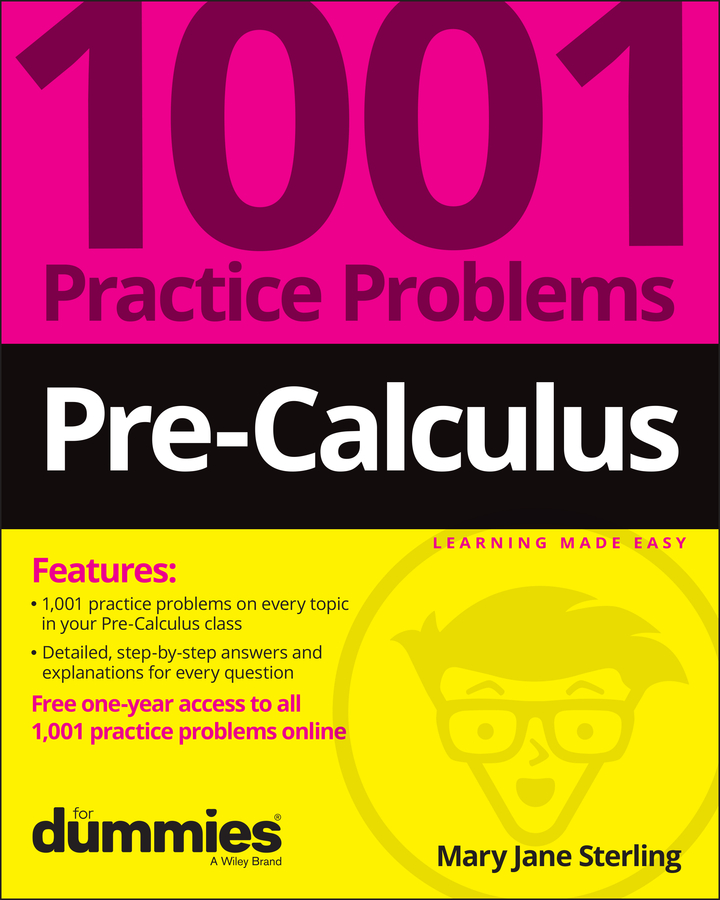An absolute-value equation usually has two possible solutions. Absolute value is a bit trickier to handle when you’re solving inequalities. The two possible solutions are:

• One where the quantity inside the absolute-value bars is greater than a number

• One where the quantity inside the absolute-value bars is less than a number

In mathematical terminology, the inequality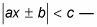where a, b, and c are real numbers — always becomes two inequalities: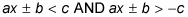The “AND” comes from the graph of the solution set on a number line, as seen in Figure (a), below. “AND” means that you want only values for x that are simultaneously solutions to both inequalities.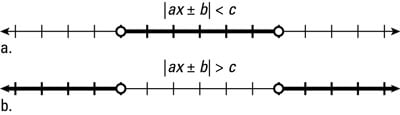The inequality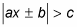becomes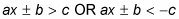The “OR” also comes from the graph of the solution set, which you can see in Figure (b), preceding. “OR” means that you want all values for x that are solutions to at least one of the inequalities.

Here are two caveats to remember when dealing with absolute values:

• If the absolute value is less than (<) or less than or equal to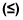a negative number, it has no solution. An absolute value must always be zero or positive (the only thing less than negative numbers is other negative numbers). For instance, the absolute-value inequality |2x – 1| < –3 doesn’t have a solution, because the inequality is less than a negative number.

Getting zero as a possible solution is perfectly fine. It’s important to note, though, that having no solutions is a different thing entirely. No solutions means that no number works at all, ever, whereas getting zero as a solution means there is a solution, namely zero.

• If the result is greater than or equal to a negative number, the solution is all real numbers. For example, given the equation |x – 1| > –5, x is all real numbers. The left-hand side of this equation is an absolute value, and an absolute value always represents a positive number (or zero). Because positive numbers (or zero) are always greater than negative numbers, these types of inequalities always have a solution. Any real number that you put into this equation works.

To solve and graph an inequality with an absolute value — for instance, 2|3x – 6| < 12 — follow these steps:

1. Isolate the absolute-value expression.

In this case, divide both sides by 2 to get |3x – 6| < 6.

2. Break the inequality in two.

This process gives you 3x – 6 < 6 and 3x – 6 > –6. Did you notice how the inequality sign for the second part changed? When you switch from positives to negatives in an inequality, you must change the inequality sign.

Don’t fall prey to the trap of changing the equation inside the absolute-value bars. For example, |3x – 6| < 6 doesn’t change to 3x + 6 < 6 or 3x + 6 > –6.

3. Solve both inequalities.

The solutions to this problem are x < 4 and x > 0. This condenses to 0.

4. Graph the solutions.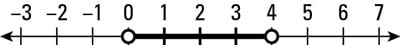Create a number line and show the answers to the inequality. The preceding figure shows the solution to 2|3x – 6| < 12 on a number line.